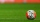# Teacher

Teacher Rem bought 360 pieces of cupcakes for the outreach program of their school. 5/9 of the cupcakes were chocolate flavor and 1/4 wete pandan flavor and the rest were vanilla flavor. How much more chocolate flavor cupcakes than vanilla flavor?

Result

x =  20

#### Solution:Leave us a comment of example and its solution (i.e. if it is still somewhat unclear...):

Showing 0 comments:Be the first to comment!#### To solve this example are needed these knowledge from mathematics:

Need help calculate sum, simplify or multiply fractions? Try our fraction calculator.

## Next similar examples:

1. Cupcakes 2Susi has 25 cupcakes. She gives 4/5. How much does she have left?
2. ZdeněkZdeněk picked up 15 l of water from a 100-liter full-water barrel. Write a fraction of what part of Zdeněk's water he picked.
3. WithdrawalIf I withdrew 2/5 of my total savings and spent 7/10 of that amount. What fraction do I have in left in my savings?
4. Fraction and a decimalWrite as a fraction and a decimal. One and two plus three and five hundredths
5. Mixed2improperWrite the mixed number as an improper fraction. 166 2/3
6. Write 2Write 791 thousandths as fraction in expanded form.
7. Fraction to decimalWrite the fraction 3/22 as a decimal.
8. Pizza 4Marcus ate half pizza on monday night. He than ate one third of the remaining pizza on Tuesday. Which of the following expressions show how much pizza marcus ate in total?
9. Lengths of the poolMiguel swam 6 lengths of the pool. Mat swam 3 times as far as Miguel. Lionel swam 1/3 as far as Miguel. How many lengths did mat swim?
10. Passenger boatTwo-fifths of the passengers in the passenger boat were boys. 1/3 of them were girls and the rest were adult. If there were 60 passengers in the boat, how many more boys than adult were there?
11. In fractionsAn ant climbs 2/5 of the pole on the first hour and climbs 1/4 of the pole on the next hour. What part of the pole does the ant climb in two hours?
12. Equation 15Solve equation with variables on both sides:
13. Jose and KaitlynJose and Kaitlyn have a contest to see who can throw a baseball the farthest. Kaitlyn wins, with a throw of 200 ft. If Jose threw the ball 3/4 as far as Kaitlyn, how far did Jose throw the ball?
14. EquationFind x: x + 1/2 = 1/3
15. Adding mixed numerals3 3/4 + 2 3/5 + 5 1/2 Show your solution.
16. Reciprocal equation 3Solve reciprocal equation: 1/2 + 2/3=1/x
17. Grandmother and grandfatherGrandmother baked cakes. Grandfather ate half, then quarter of the rest ate Peter and Paul ate half of rest. For parents left 6 cakes. How many cakes maked the grandmother?